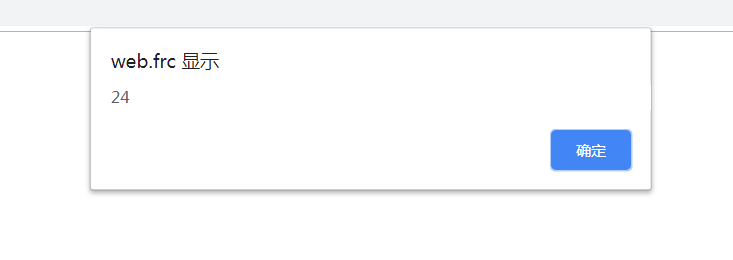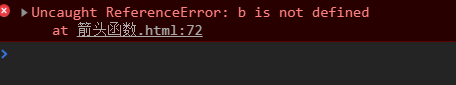﻿ 聊聊 ES6 中的箭头函数-前端-比特在线

### 聊聊 ES6 中的箭头函数

1. 当只有一个参数的时候，那么 () 可以省略
2. 当只有一个 return 的时候，那么 {} 可以省略
3. 当函数体内只有一条语句的时候，那么 {} 也可以省略

ES5:

```        window.onload = function () {
}```

ES6:

```window.onload = () => {
}```

ES5:

```        let show = function (a, b) {
}
show(2, 3);```

ES6:

```        let show = (a, b) => {
}
show(2, 3);```

```        let show = a => {
return a * 2;
}```        let show = a => return a * 2;

```        let show = a => a * 2;

```        let show = (a, b) => console.log(a + b);
show(1, 2);```

```        let show = (a, b) => a = a.toString(); b = b.toString(); console.log(a + b);
show(1, 2);``````        let show = (a, b) => {a = a.toString(); b = b.toString(); console.log(a + b)};
show(1, 2);// => 3```